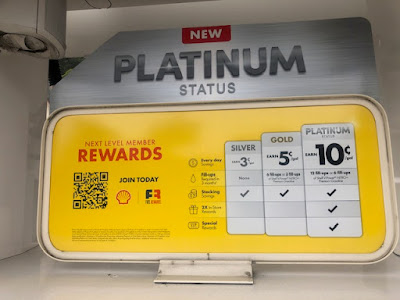## February 03, 2022

### Delayed Impact

When a business is stuck, you witness the slow death of the customer file. Here's an example.

Let's assume that we have a burst in new customers, from 462,682 in Year 1 to 600,000. What happens to the customer file over time?

• Year 0 = 88,437.
• Year 1 = 86,789.
• Year 2 = 84,379.
• Year 3 = 82,679.
• Year 4 = 81,411.
• Year 5 = 80,577.
And this is what it looks like with a major bump in new customers.
• Year 0 = 88,437.
• Year 1 = 86,789.
• Year 2 = 91,067.
• Year 3 = 86,966.
• Year 4 = 84,960.
• Year 5 = 83,170.
Here's the difference by year.
• Year 0 = 0.
• Year 1 = 0.
• Year 2 = 6,688.
• Year 3 = 4,287.
• Year 4 = 3,549.
• Year 5 = 2,593.
The payoff comes downstream. You acquire a lot more customers today, and in years two through five you have more outstanding customers who deliver disproportionate profit. There's a delayed impact at the top of the file.

If you want loyal customers tomorrow, acquire more customers today.